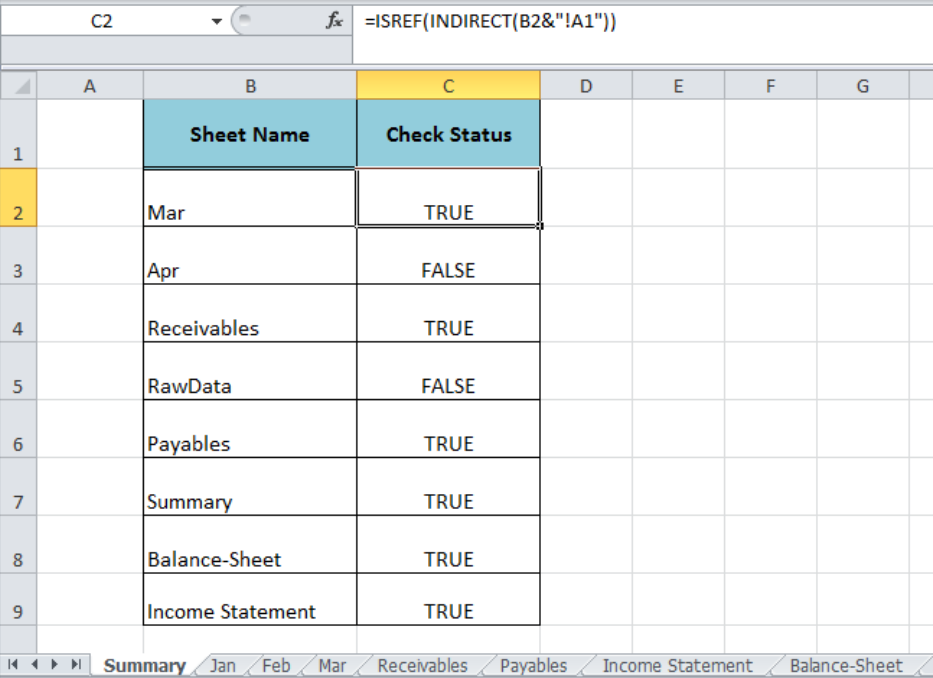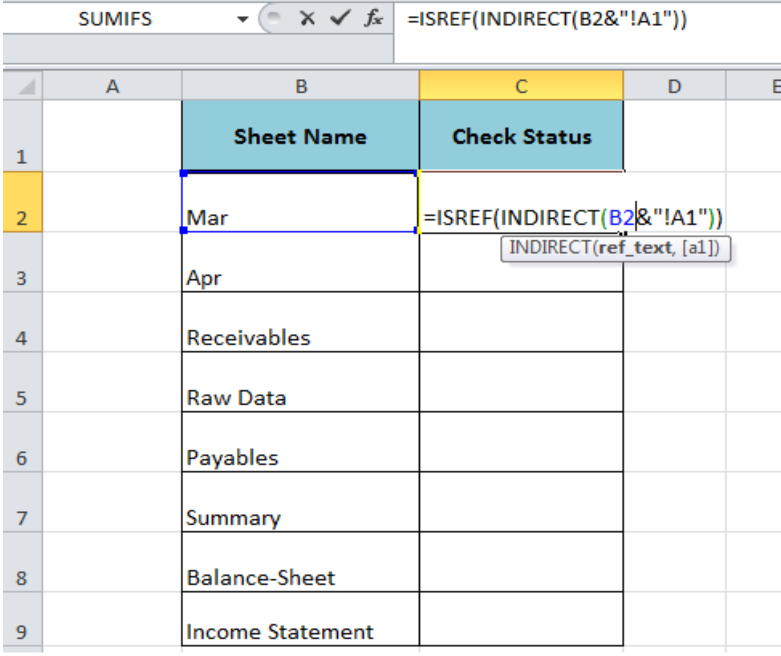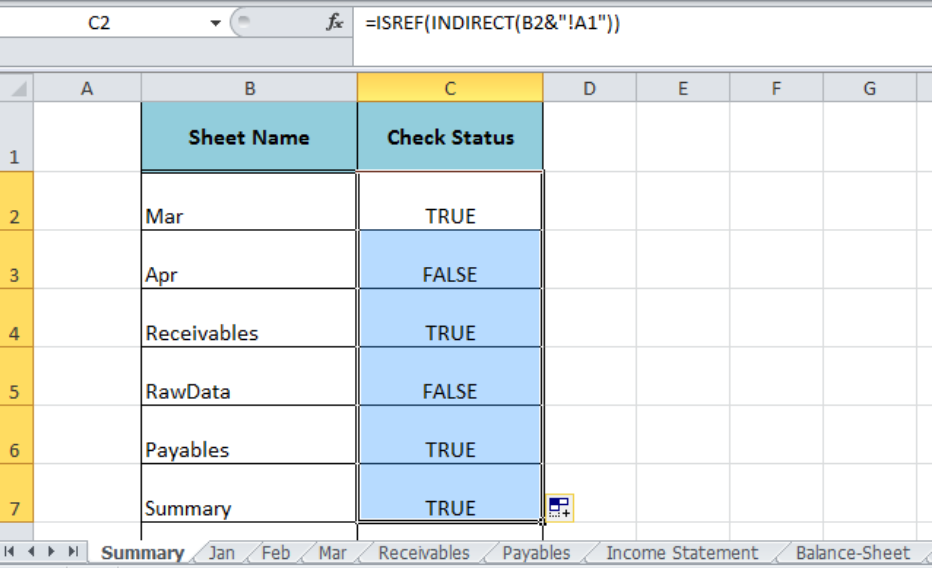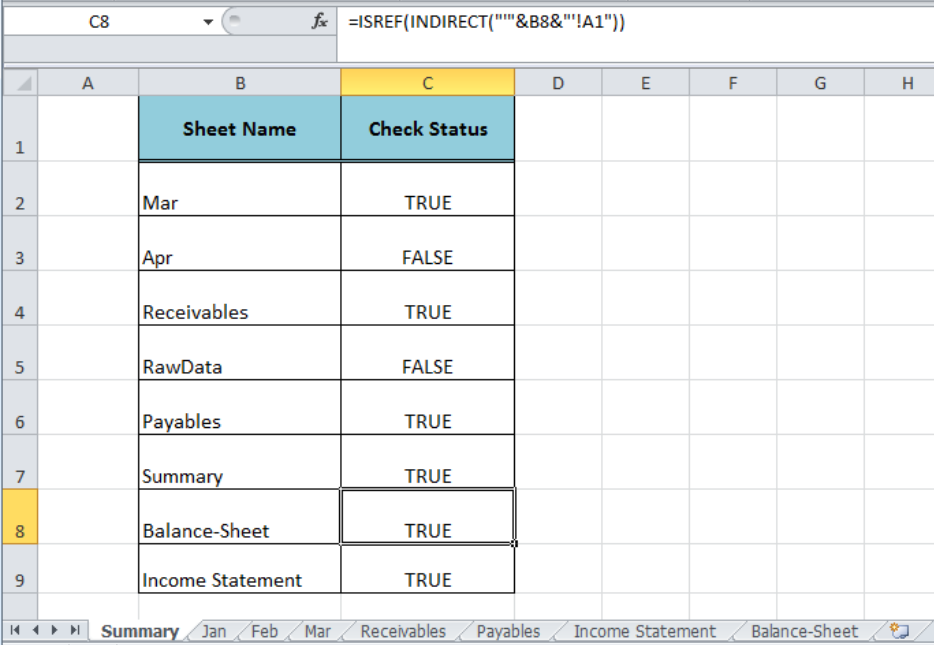Get instant live expert help with Excel or Google Sheets“My Excelchat expert helped me in less than 20 minutes, saving me what would have been 5 hours of work!”

#### Post your problem and you’ll get expert help in seconds.

Your message must be at least 40 characters
Our professional experts are available now. Your privacy is guaranteed.

# How to Check If Worksheet Name Exists in a Workbook

In order to check whether a certain worksheet name exists in a workbook or not, we can perform this check with the help of the Excel ISREFand INDIRECT functions. This article demonstrates how to perform this.Figure 1. Checking Worksheet Name in a Workbook

## Formula Syntax

The generic formula syntax to check the worksheet name in a workbook is;

`=ISREF(INDIRECT("sheetname"&"!A1"))`

The Excel ISREF function checks whether a value is a reference and returns TRUE or FALSE and the INDIRECT function returns the reference specified by a text string. In this formula, we can supply the worksheet name as a text value or cell reference and create a full reference of the worksheet name we combine it with an exclamation mark and a general cell reference, such as “!A1”, using the Ampersand symbol (&).Figure 2. The Syntax of the Formula

## Worksheet Name Without Spaces Or Punctuation

The above generic syntax of the formula checks the status of a worksheet name that is without spaces or punctuation in a workbook, like “Mar” or “Payables” etc. Suppose we have different worksheet names in column B that do not contain any space or punctuation and want to check if a worksheet name exists in a workbook. In this case, we will use the following formula in cell C2, such as;

`=ISREF(INDIRECT(B2&"!A1"))`

Copy down this formula to return the results for all the listed names of worksheets.Figure 3. Checking the Worksheet Name Without Spaces Or Punctuation

## Worksheet Name With Spaces Or Punctuation

If a worksheet name contains spaces or punctuation, like “Income Statement” and “Balance-Sheet” etc, then we need to amend the generic syntax of formula a little bit. To handle this, we will wrap the worksheet name in the single quotation mark as per the following formula syntax in cell C8 or C9;

`=ISREF(INDIRECT(" ' "&sheetname&" ' !A1"))`

`=ISREF(INDIRECT("'"&B8&"'!A1"))`Figure 4. Checking the Worksheet Name With Spaces Or Punctuation

## Instant Connection to an Expert through our Excelchat Service:

Most of the time, the problem you will need to solve will be more complex than a simple application of a formula or function. If you want to save hours of research and frustration, try our live Excelchat service! Our Excel Experts are available 24/7 to answer any Excel question you may have. We guarantee a connection within 30 seconds and a customized solution within 20 minutes.

### Did this post not answer your question? Get a solution from connecting with the expert.Another blog reader asked this question today on Excelchat:
Solution examplesUse the Vlookup Function to complete the "employee" column of table 2. Use "job Id" from table 2 as your lookup_value(s) and table 1 as your reference.
Solved by C. H. in 16 minsIf a cell in another sheet is populated I need a vlookup done. If the cell is not populated I need the cell to return blank.
Solved by T. D. in 60 minsI am trying to make a chart that turns a week range red if nothing is entered in the range. If something is entered then I would like it to turn green. Please Help
Solved by E. U. in 43 minsI need a check box to show/hide an answer of an if function
Solved by Z. U. in 23 minsI need a formula to compare the data in two columns and then export the mismatched data in the 3rd column
Solved by S. Q. in 20 mins## Subscribe to Excelchat.coAnother blog reader asked this question today on Excelchat: